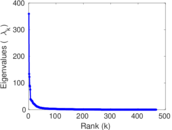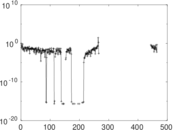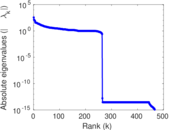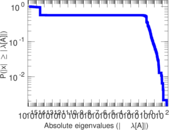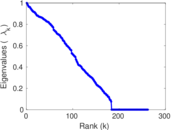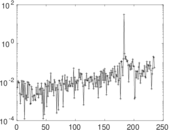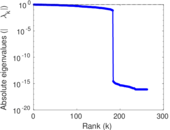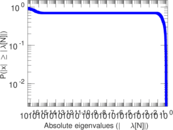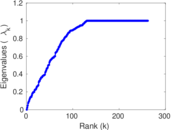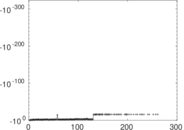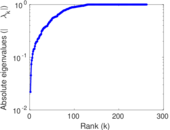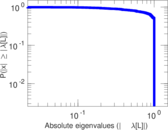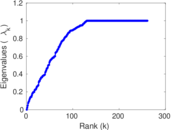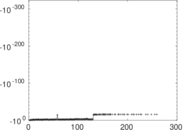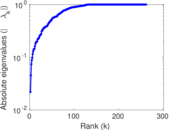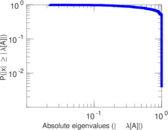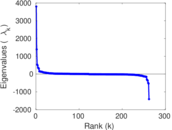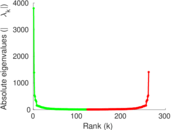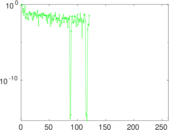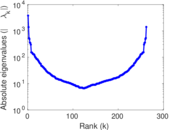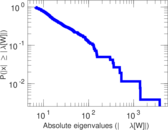# Wikiquote edits (te)

This is the bipartite edit network of the Telugu Wikiquote. It contains users and pages from the Telugu Wikiquote, connected by edit events. Each edge represents an edit. The dataset includes the timestamp of each edit.

 Code `qte` Internal name `edit-tewikiquote` Name Wikiquote edits (te) Data source http://dumps.wikimedia.org/ AvailabilityDataset is available for download Consistency checkDataset passed all tests Category Authorship network Dataset timestamp 2017-10-20 Node meaning User, article Edge meaning Edit Network formatBipartite, undirected Edge typeUnweighted, multiple edges Temporal dataEdges are annotated with timestamps

## Statistics

 Size n = 3,795 Left size n1 = 468 Right size n2 = 3,327 Volume m = 11,174 Unique edge count m̿ = 4,982 Wedge count s = 2,127,490 Claw count z = 1,339,876,784 Cross count x = 666,337,117,216 Square count q = 37,827 4-Tour count T4 = 8,823,092 Maximum degree dmax = 4,796 Maximum left degree d1max = 4,796 Maximum right degree d2max = 308 Average degree d = 5.888 80 Average left degree d1 = 23.876 1 Average right degree d2 = 3.358 58 Fill p = 0.003 199 67 Average edge multiplicity m̃ = 2.242 87 Size of LCC N = 3,515 Diameter δ = 12 50-Percentile effective diameter δ0.5 = 3.302 62 90-Percentile effective diameter δ0.9 = 5.309 29 Median distance δM = 4 Mean distance δm = 3.714 15 Gini coefficient G = 0.786 166 Balanced inequality ratio P = 0.173 573 Left balanced inequality ratio P1 = 0.104 976 Right balanced inequality ratio P2 = 0.242 796 Relative edge distribution entropy Her = 0.761 781 Power law exponent γ = 4.746 24 Tail power law exponent γt = 2.561 00 Tail power law exponent with p γ3 = 2.561 00 p-value p = 0.000 00 Left tail power law exponent with p γ3,1 = 1.861 00 Left p-value p1 = 0.410 000 Right tail power law exponent with p γ3,2 = 2.811 00 Right p-value p2 = 0.000 00 Degree assortativity ρ = −0.324 786 Degree assortativity p-value pρ = 9.848 55 × 10−123 Spectral norm α = 359.345 Spectral separation |λ1[A] / λ2[A]| = 2.682 03 Controllability C = 3,216 Relative controllability Cr = 0.858 516

## Plots

### Fruchterman–Reingold graph drawing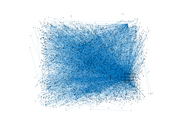### Degree distribution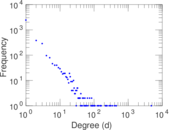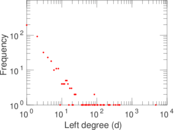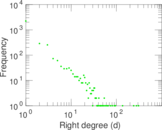### Cumulative degree distribution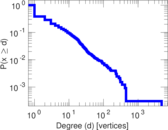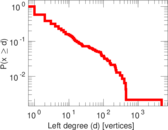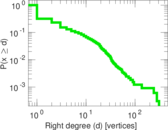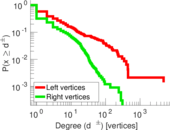### Lorenz curve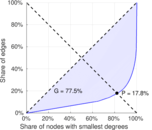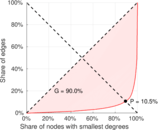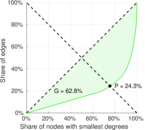### Spectral distribution of the adjacency matrix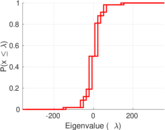### Spectral distribution of the normalized adjacency matrix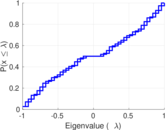### Spectral distribution of the Laplacian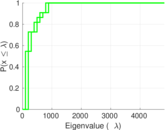### Spectral graph drawing based on the adjacency matrix### Spectral graph drawing based on the Laplacian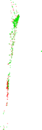### Spectral graph drawing based on the normalized adjacency matrix### Degree assortativity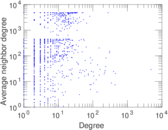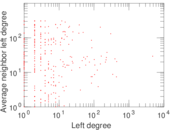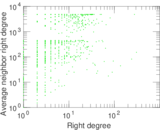### Zipf plot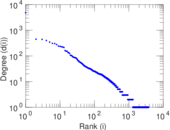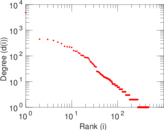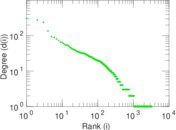### Hop distribution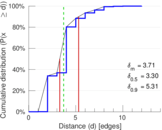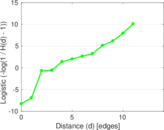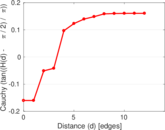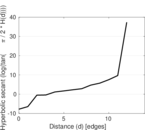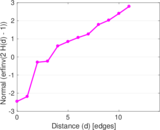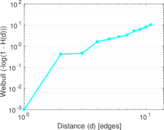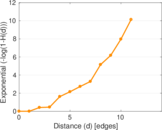### Double Laplacian graph drawing### Delaunay graph drawing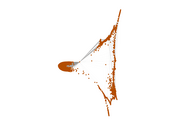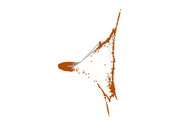### Edge weight/multiplicity distribution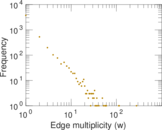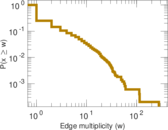### Temporal distribution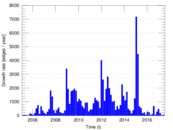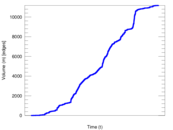### Temporal hop distribution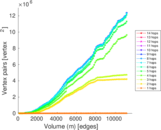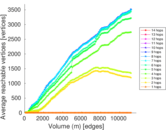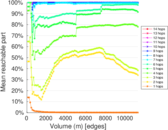### Diameter/density evolution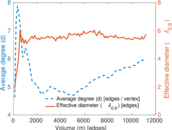### Matrix decompositions plots